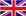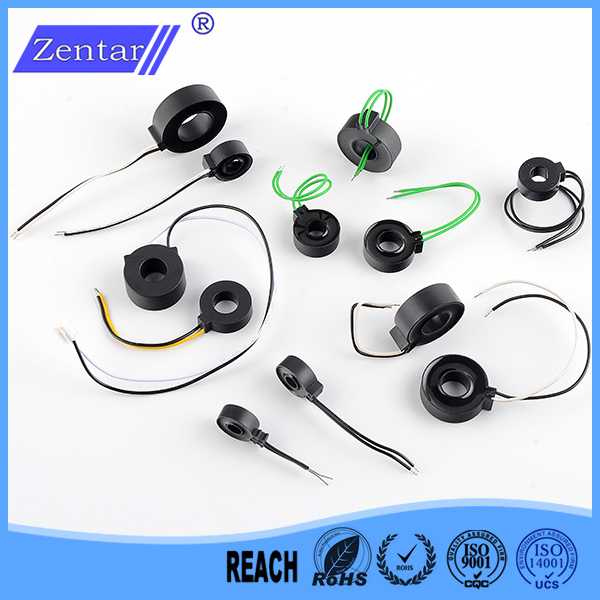English
Blog

### The difference between current transformer and zero sequence current transformer

June 09 , 2020
1. The basic function of current transformers

The current transformers can convert primary currents with larger values to secondary currents with lower values through a certain transformation ratio, which are used for protection and measurement purposes. For example, a current transformer with a transformation ratio of 400/5 can convert the actual 400A current to 5A current.

2. Working principle of zero sequence current transformer

The basic principle of zero-sequence current protection is based on Kirchhoff's current law: the algebraic sum of the complex current flowing into any node in the circuit is equal to zero. When the line and electrical equipment are normal, the vector sum of each phase current is equal to zero. Therefore, the secondary winding of the zero sequence current transformer has no signal output, and the actuator does not operate. When the ground fault occurs, the vector sum of each phase current is not zero. The fault current causes magnetic flux in the ring core of the zero-sequence current transformer. The induced voltage on the secondary side of the zero-sequence current transformer causes the actuator to move and drive Trip device to switch the power supply network to achieve the purpose of ground fault protection.

3. The function of zero sequence current transformer

When an electric shock or leakage fault occurs in the circuit, the protection acts and the power supply is cut off.

4. Conditions of use for zero sequence current transformers

A current transformer can be installed on each of the three-phase lines, or three-phase conductors can be passed through a zero-sequence current transformer together, or a zero-sequence current transformer can be installed on the neutral line N to use it to detect three phases. Vector sum of current.

For the specific application of zero-sequence current protection, a current transformer (CT) can be installed on each of the three-phase lines, or three-phase conductors can be passed through a zero-sequence CT together, or a zero-sequence CT can be installed on the neutral line N. These CTs are used to detect the vector sum of three-phase current, that is, the zero-sequence current Io, IA+IB+IC=IO, when the three-phase load connected to the line is fully balanced (no ground fault, and the leakage current of the line and electrical equipment is not considered ), IO=0; when the three-phase load connected to the line is unbalanced, then IO=IN, and the zero-sequence current at this time is the unbalanced current IN; when a ground fault occurs in a phase, a single-phase ground must be generated The fault current Id, the zero sequence current detected at this time IO=IN+Id, is the vector sum of the three-phase unbalanced current and the single-phase ground current.

Current transformer manufacturer and experts#### Tags

welcome to ZTC
Talk To ZTC Today To Discuss Your Need For Current Transformers,Get Standard Item, OEM/ODM Service Quote Are Available.